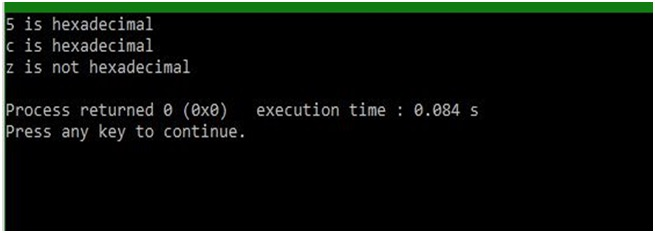# isxdigit() function of ctype.h in C

In this article, we are going to learn about the isxdigit() function of ctype.h header file in C programming language and use it with the help of an example.
Submitted by Abhishek Sharma, on April 11, 2018

This function is used to check if the character is hexadecimal or not, the Function returns 1 if the character is hexadecimal and 0 if the character is not hexadecimal.

The Hexadecimal characters are typically in the range of,

1. 0 - 9
2. A - F
3. a - f

Anything else other than this is not hexadecimal.

Example:

```    Input character is: 'a'
Function will return 1

Input character is: 'Z'
Function will return 0
```

## ctype.h - isxdigit() function Example in C

﻿

```#include <stdio.h>
#include <ctype.h>

int main()
{
// defining the type of variable
char a,b,c;

// assigning the values of variables
a = '5';
b = 'c';
c = 'z';

// Condition to check, if the value of variable is hexadecimal digit
if (isxdigit(a))
{
}
else
{
}

// Condition to check, if the value of variable is hexadecimal digit
if (isxdigit(b))
{
}
else
{
}

// Condition to check, if the value of variable is hexadecimal digit
if (isxdigit(c))
{
}
else
{
}

return 0;
}
```

Output